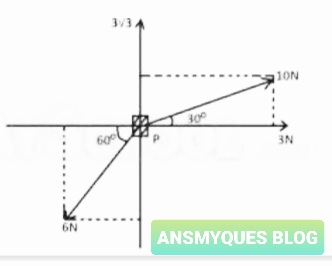# PHYSICS QUESTIONS AND ANSWERS (5)

1. If a spherical metal bob of radius 3cm is fully immersed in a cylinder containing water and the water level rises by 1cm, what is the radius of the cylinder?
A. 12cm
B. 1cm
C. 3cm
D. 6cm

77

2. The resultant of two forces acting on an object is maximum if the angle between them is
A. 45°
B. 0°
C. 90°
D. 180°

3. Which of the following consists entirely of vector quantities?
A. Velocity, magnetic flux and reaction
B. Tension, magnetic flux and mass
C. Displacement, impulse and power
D. Work, pressure and moment

4. The component of vector X along the direction of vector Y is zero when
A. X and Y are in opposite directions to each other
B. X is at an angle of 45° to Y
C. X is perpendicular to Y
D. X and Y are parallel to each other

5. The correct unit of energy density is?
A. kg m
3 s-2
B. kg m
2 s-2
C. kg m
-3 s-2
D. kg m
-1 s-2

6. Two forces of magnitudes 7N and 3N act at right angles to each other. The angle θ between the resultant and the 7N force is given by
A. cos
θ = 3/7
B. sin
θ = 3/7
C. tan
θ = 3/7
D. cot
θ = 3/7

7. The dimension of electromotive force are `
A. ML
2T-3I-1
B. ML
2T-3I-2
C. M
2LT-2I-1
D. M
2L2T-1I-1

558

8. The resultant of two forces 12N and 5N is 13N. What is the angle between the two forces?
A. 0
o
B. 45
o
C. 90
o
D. 180
o

9. An air craft attempt to fly due north at 100 kmh-1. If the wind blows against it from east to west at 60 kmh-1, its resultant velocity is
A. 117 kmh
-1, N31oE
B. 127 kmh
-1, N31oE
C. 117 kmh
-1, N31oW
D. 127 kmh
-1, N31oW

10. I Electrical potential, II Torque, III Kinetic Energy, IV Momentum. Which of the quantities listed are vectors?
A. II and IV
B. I and II
C. I and III
D. II and III

11. At what respective values of x, y and z would the unit of force, the newton, be dimensionally equivalent to MxLyTz?
A. -1, 1, 2
B. 1, 1, -2
C. 1, -1, 2
D. -1, 1, -2
76912. The figure above shows 4 forces 3N, 10N, 3√3N and 6N acting on a particle P. The resultant of the four forces is
A. 5N
B. 5√ 3N
C. 10N
D. 10√ 3N

13. One of the following readings represents the measurement of the length of a metal rod using Vernier calipers, Taking the reading accuracy into consideration, the most likely one is
A. 5.15cm
B. 5.165cm
C. 5.0cm
D. 5.160cm

14. Which of the following is a fundamental unit?
A. Newton
B. Watt
C. Joule
D. Second

15.Which of the following is a vector?
A. Electric charge
B. Electric field
C. Electrical potential difference
D. Electrical capacitance

16. Which of the following are the correct SI units of the quantities indicated?

i. N(Force) ii. Nm-1 (Torque)

iii. watt(Power) iv. Kgms-2 (work)
A. i and ii only
B. i, ii and iii only
C. i, ii, and iv only
D. i and iii only
1083

17. A lorry travels 10km northwards, 4km eastwards, 6km southwards, 4km westwards to arrive at a point T. What is the total displacement?
A. 6km south
B. 4km north
C. 6km north
D. 4km east
1084

18. Two forces whose resultant is 100N, are at right angle to each other. If one of them makes an angle 30o with the resultant, determine its magnitude.
A. 8.66N
B. 50.0N
C. 57.7N
D. 86.6N
1085

See also  The expansion of white communities in South Africa is a major cause of ________

19. Which of the following quantities are scalars?

i. Electrical potential  ​​ ​​ ​​ ​​ ​​ ​​​​ ii. Torque  ​​ ​​ ​​ ​​ ​​ ​​ ​​ ​​ ​​ ​​ ​​ ​​ ​​​​ iii. Momentum  ​​ ​​ ​​ ​​ ​​ ​​ ​​​​ iv. Kinetic energy
A. ii and iii only
B. i and ii only
C. iii and iv only
D. i and iv only

20. Which of the following represents the correct precision if the length of a piece of wire is measured with a meter rule?
A. 35mm
B. 35.0mm
C. 35.00mm
D. 35.01mm

you can use the EDUCATION CATEGORY to find more physics, chemistry and other questions with answer…good luck to us all, do well to keep coming back because that’s the motive behind the name of this site ANSMYQUES (ANSWER MY QUESTION). Or equally you can use the search box above or below TYPE PHYSICS and it will bring out all physics questions on this plattform, this idea can be use for other subjects too.